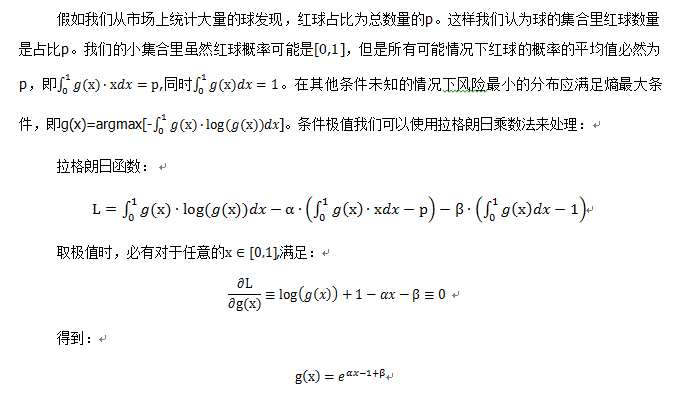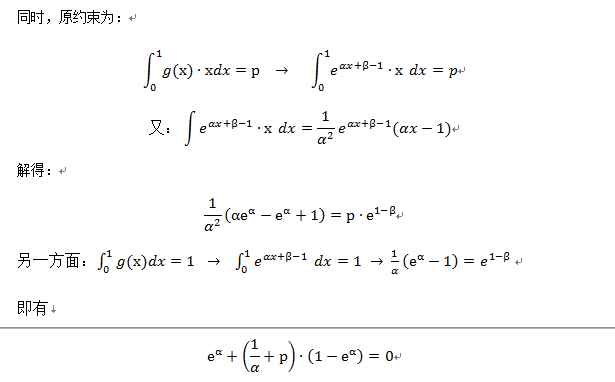# 小样本分析（三）

我怀着日思夜想的问题，希望你能给我答案，你慷慨的给与我答案，又慷慨的给了我一个新的问题。【注：拉格朗日乘数法我也忘记得差不多了，在网上找到的例子也主要是只有一个约束条件，这里到两个约束的拓展有点不确定，但是先做了，后面也得到了想要的结果，这里先不管了】# coding=utf-8
__author__ = '01053185'
import numpy as np
import math

def error_f(alpha, p):
# 计算误差的绝对值 e^α+(1/α+p)∙(1-e^α)=0  方程
return abs(math.exp(alpha) + (1.0 / alpha + p) * (1 - math.exp(alpha)))

class P_test():
def __init__(self):
self.alpha = 0
self.beta = 0
self.p_pre = 0
self.x_array = np.array([0.0] * 10000)
self.pro_x_array = np.array([0.0] * 10000)
self.step_x_array = np.array([0.0] * 10000)
self.p_estimate = 0  # 最后估计的概率值

def solve_function(self, p):
self.p_pre = p
alpha = -1000 + 10 ** -9
sign = 1.0
step = 1.0
error_now = error_f(alpha, self.p_pre)
T = 0
while (error_now > 10 ** -9):
temp_alpha = alpha + step * sign
temp_error = error_f(temp_alpha, self.p_pre)
if temp_error < error_now:
alpha = temp_alpha
error_now = temp_error
T += 1
if T == 2:
step *= 2
continue
sign *= -1  # 反向尝试
temp_alpha = alpha + step * sign
temp_error = error_f(temp_alpha, self.p_pre)
if temp_error < error_now:
T = 0
alpha = temp_alpha
error_now = temp_error
continue
step *= 0.3
self.alpha = alpha
self.beta = 1 - math.log(1.0 / self.alpha * (math.exp(self.alpha) - 1))

def set_array(self):
start = 0.0
step = 10.0 ** -9
x_array = np.array([0.0] * 100000)  # 记录某个区间的中间点
pro_x_array = np.array([0.0] * 100000)  # 记录某个区间的概率
step_x_array = np.array([0.0] * 100000)  # 记录某个区间的宽度
step_i = 0
while start < 1:
# print step
temp_x = 0.5 * (start + min(start + step, 1))
step_end = min(start + step, 1)
temp_step_x = step_end - start
# 概率密度为：math.exp(self.alpha * temp_x - 1 + self.beta) 取其对数
temp_pro = self.alpha * temp_x - 1 + self.beta
if math.exp(temp_pro)*temp_step_x < 0.00001 and step<0.0005:
step *= 2
continue
else:
x_array[step_i] = temp_x
step_x_array[step_i] = temp_step_x
pro_x_array[step_i] = temp_pro
start =  step_end
step_i += 1
self.x_array = x_array[0:step_i]  # 每种情况的发生概率 以该区间的中值为准
self.pro_x_array = pro_x_array[0:step_i]  # 每种情况的对数概率值
self.step_x_array = step_x_array[0:step_i]  # 每种情况的取值区间大小

def get_pro(self, n, m):
# 计算不同的段x概率在m次试验中,发生n次的概率  使用对数避免精度损失
# self.pro_x_array * self.step_x_array 每种情况发生的原始分布
now_jiashe = np.log(self.x_array) * n + np.log(1 - self.x_array) * (m - n) + self.pro_x_array + np.log(self.step_x_array)  # 调整原始的经验分布
my_max = now_jiashe.max()  # 以最大值为1 进行计算
now_jiashe = now_jiashe - my_max
now_jiashe = np.exp(now_jiashe)/sum(np.exp(now_jiashe))  # 返回为不同情况的 真实的概率分布
return sum(now_jiashe * self.x_array)

if __name__ == "__main__":
a = P_test()
a.solve_function(0.001)  #初始经验概率
# print sum(a.array_pro_pro)
a.set_array()
print a.get_pro(0,0) #0次抽样，0次发生
print a.get_pro(10000,10000)  #10000次抽样，10000次发生


solve_function这个函数是利用随机行走的办法找方程的解，原方程有一个0伪解（alpha趋于0时，方程的误差也趋于零），这里通过初始设置避开了，找到那个非零解。

error_f 则是计算某个尝试解与方程的符合程度。这个里面真正的包含了需要求解的方程

set_array 这个函数则是知道概率密度分布函数后，将其划分为0-1区间各种不同概率的离散情况便于数值计算。这个划分（离散化）方法直接会影响到后面拟合概率的精度。精度的控制由 math.exp(temp_pro)*temp_step_x < 0.00001 and step<0.0005 这条判断语句限定，前者是对概率区间的初始概率进行限制，后者是对概率区间的大小进行限制。总之最后得到概率在0-1之间各种不同情况发生的概率.（精度原因都取了对数），也就是先验概率分布。

get_pro 则是结合m次抽样，发生n次之后这一事件，计算后验概率密度分布，最后返回该概率分布下的概率期望。

07-03283

#### 小样本04-032万+

#### 小样本学习(one/few-shot learning)

05-31445

#### 小样本学习

05-141369

#### 小样本学习知识点汇总

12-307万+

#### 当小样本遇上机器学习 fewshot learning

04-011万+

#### 小样本学习（Few-shot Learning）综述

03-134248

#### 小样本学习之半监督的小样本分类©️2020 CSDN 皮肤主题: 编程工作室 设计师: CSDN官方博客点击重新获取扫码支付1.余额是钱包充值的虚拟货币，按照1:1的比例进行支付金额的抵扣。
2.余额无法直接购买下载，可以购买VIP、C币套餐、付费专栏及课程。余额充值